# Arakelov geometry

(diff) ← Older revision | Latest revision (diff) | Newer revision → (diff)

Arakelov theory

A combination of the Grothendieck algebraic geometry of schemes overwith Hermitian complex geometry on their set of complex points. The goal is to provide a geometric framework for the study of Diophantine problems in higher dimension (cf. also Diophantine equations, solvability problem of; Diophantine problems of additive type).

The construction relies upon the analogy between number fields and function fields: the ring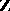has Krull dimension (cf. Dimension) one, and "adding a point"to the corresponding schememakes it look like a complete curve. For instance, if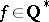is a rational number, the identity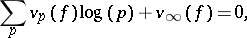whereis the valuation ofat the primeand where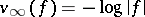, is similar to the Cauchy residue formula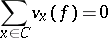for the differential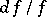, whenis a non-zero rational function on a smooth complex projective curve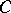.

In higher dimension, given a regular projective flat schemeover, one considers pairs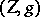consisting of an algebraic cycle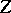of codimensionover, together with a Green current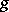foron the complex manifold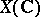:is real current of type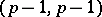such that, if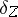denotes the current given by integration on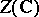, the following equality of currents holds:whereis a smooth form of type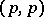. Equivalence classes of such pairsform the arithmetic Chow group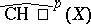, which has good functoriality properties and is equipped with a graded intersection product, at least after tensoring it by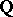.

These notions were first introduced for arithmetic surfaces, i.e. models of curves over number fields [a1], [a2] (for a restricted class of currents). For the general theory, see [a7], [a9] and references therein.

Given a pairconsisting of an algebraic vector bundle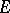onand a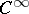Hermitian metricon the corresponding holomorphic vector bundle on the complex-analytic manifold, one can define characteristic classes of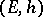with values in the arithmetic Chow groups of. For instance, whenhas rank one, ifis a non-zero rational section ofand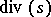its divisor, the first Chern class ofis the class of the pair. The main result of the theory is the arithmetic Riemann–Roch theorem, which computes the behaviour of the Chern character under direct image [a8], [a6]. Its strongest version involves regularized determinants of Laplace operators and the proof requires hard analytic work, due to J.-M. Bismut and others.

Since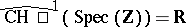, the pairings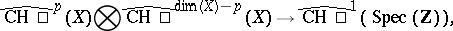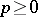, give rise to arithmetic intersection numbers, which are real numbers when their geometric counterparts are integers. Examples of such real numbers are the heights of points and subvarieties, for which Arakelov geometry provides a useful framework [a3].

Whenis a semi-stable arithmetic surface, an important invariant ofis the self-intersection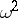of the relative dualizing sheaf equipped with the Arakelov metric [a1]. L. Szpiro and A.N. Parshin have shown that a good upper bound forwould lead to an effective version of the Mordell conjecture and to a solution of the abc conjecture [a10]. G. Faltings and E. Ullmo proved thatis strictly positive [a4], [a11]; this implies that the set of algebraic points ofis discrete in its Jacobian for the topology given by the Néron–Tate height.

P. Vojta used Arakelov geometry to give a new proof of the Mordell conjecture [a12], by adapting the method of Diophantine approximation. More generally, Faltings obtained by Vojta's method a proof of a conjecture of S. Lang on Abelian varieties [a5]: Assumeis an Abelian variety over a number field and let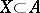be a proper closed subvariety in; then the set of rational points ofis contained in the union of finitely many translates of Abelian proper subvarieties of.